# Natural convection and entropy production in a cubic cavity heated via pin-fins heat sinks

Natural convection and entropy production in a cubic cavity heated via pin-fins heat sinks

Abdullah A.A.A. Al-Rashed Lioua KolsiHakan F. Ozto Nidal Abu-Hamdeh Mohamed N. Borjini

Dept. of Automotive and Marine Engineering Technology, College of Technological Studies, the Public Authority for Applied Education and Training, Kuwait

College of Engineering, Mechanical Engineering Department, Haïl University, Haïl City, Saudi Arabia

Unité de Métrologie et des Systèmes Énergétiques, École Nationale d’Ingénieurs, Monastir, University of Monastir, Tunisia

Department of Mechanical Engineering, Technology Faculty, Fırat University, Elazig, Turkey

Department of Mechanical Engineering, King Abdulaziz University, Jeddah, Saudi Arabia

Corresponding Author Email:
lioua_enim@yahoo.fr
Page:
109-115
|
DOI:
https://doi.org/10.18280/ijht.350115
|
Accepted:
|
Published:
31 March 2017
| Citation

OPEN ACCESS

Abstract:

In this study, heat transfer, flow structure and produced entropy due to natural convection in a three-dimensional cavity heated via heat sinks are investigated numerically. One wall of the cavity is heated via pin-fins and opposite wall is maintend at lower temperature. Remaining bouandaries are considered as adiabatic. Finite volume method is used to solve governing equations. Three geometrical cases are tested according to number and location of the fins. Other governing parameters are Rayleigh number and fin length. Number and length of the fins were found to be the most effective parameters on both heat transfer and entropy generation.

Keywords:

Entropy Production, 3D Natural Convection, Heat Sinks, Flow Structure

1. Introduction

Heated pins are used for different application areas in engineering such as passive cooling or heating of rooms, buildings, radiators, heat exchangers, boilers and some solar applications. In these systems, the predominant heat transfer mechanism is natural convection. Calculation of generated entropy is very important to make good design and energy efficient systems [1-6].

An experimental comparison study has been done by Kim et al.  to compare efficiencies of plate-fin and pin-fin heat sinks. Results indicate better performances of plate-fin.   Yalcın et al.  studied the three-dimensional heat transfer by testing the clearance gap between fin tips and shroud. The heat transfer was found to increase proportionally to the clearance parameter. Varol et al.  worked on isothermal longitudinal heater located in triangular enclosure. They observed that taller cavity and central position of the fin give higher heat exchange. Appadurai and Velmurugan  used fins to improve the performances of a solar still. They conducted both theoretical and experimental work to compare conventional still and different types of finned stills. The heat transfer was increased by attaching fins. Fins were also used for heat exchangers as given by Ryu and Lee . Joo and Kim  studied the heat transfer of a vertical plate equipped by plate-fin or pin-fin heat sinks. They developed a correlation which they validated experimentally.

Research studies on 3D natural convection are very few. Three-dimensional natural convection in a plate-type fin attached surface was analyzed by Baskaya et al.  using a commercial code. They developed a correlation between geometrical parameters and Rayleigh number to estimate the rate of heat transfer. They found that the increase of fin height enhances the heat transfer. Da Silva and Gosselin  studied the 3D natural convection in cubic cavity equipped by a conductive fin. They showed that the geometrical parameters have an important effect on heat transfer and flow structure. Bocu and Altac  conducted a three-dimensional study on free convection heat transfer and fluid flow with pin-fin arrays. They showed heat transfer varies proportionally to Rayleigh number. Recently Kolsi et al [16-22] published some paper on natural convection in 3D cavities with inside different shapes active and non-active obstacles.

In this work the natural convection in a cubic cavity heated by circular fins is exanimated numerically to study heat transfer, flow structure and entropy generation with a focus on the fins number and length.

2. Physical Model

Figure 1 shows a three-dimensional isometric configuration (on the left) and a section of this configuration (in the middle). Three different configurations are chosen and named as Cases

I, II and III as shown at the right side of Figure 1. As shown on the figure, heated pins are mounted on the left hot wall and the right-side wall is maintained at cold temperature. All other walls are considered as adiabatic and gravity acts in y-direction.​

## 1.pngFigure 1. Physical Model; (a): 3D configuration; (b): sectional plan; (c): cases

3. Governing Equations and Numerical Procedure

The formulation $(\vec{\psi }-\vec{\omega })$ is used for the numerical model. This Formalism is defined by the two following relations:

$\vec{\omega }'=\vec{\nabla }\times \vec{V}'$ and $\vec{V}'=\vec{\nabla }\times \vec{\psi }'$.

The setting for the above relations exists with more details in the work of Kolsi et al. . The dimensionless governing equations are as follow:

$-\vec{\omega }={{\nabla }^{2}}\vec{\psi }$          (1)

$\frac{\partial \vec{\omega }}{\partial t}+(\vec{V}.\nabla )\vec{\omega }-(\vec{\omega }.\nabla )\vec{V}=\Delta \vec{\omega }+Ra.\Pr .\left[ \frac{\partial T}{\partial z};0;-\frac{\partial T}{\partial x} \right]$           (2)

$\frac{\partial T}{\partial t}+\text{ }\vec{V}.\nabla T=\Delta T$         (3)

With $\Pr ={\nu }/{\alpha }\;$ and $Ra=\frac{g.\beta .\Delta T.{{l}^{3}}}{\nu .\alpha }$, the considered boundary conditions are:

temperature

T=1 at x=0 and in the fins, T=0 at

$\frac{\partial T}{\partial n}=0$ on all other walls.

velocity

${{V}_{x,y,z}}=0$ on all walls

The generated entropy is written in the following form:

$S{{'}_{gen}}=\frac{1}{T{{'}^{2}}}.\vec{q}.\vec{}T'+\frac{\mu }{T'}.\varphi '$          (4)

with $\vec{q}=-k.gra\vec{d}T$

The dissipation function Φ’ is written in incompressible flow as:

\begin{align} & \varphi '=2\left[ {{\left( \frac{\partial V{{'}_{x}}}{\partial x'} \right)}^{2}}+{{\left( \frac{\partial V{{'}_{y}}}{\partial y'} \right)}^{2}}+{{\left( \frac{\partial V{{'}_{z}}}{\partial z'} \right)}^{2}} \right] \\ & +{{\left( \frac{\partial V{{'}_{y}}}{\partial x'}+\frac{\partial V{{'}_{x}}}{\partial y'} \right)}^{2}}+{{\left( \frac{\partial V{{'}_{z}}}{\partial y'}+\frac{\partial V{{'}_{y}}}{\partial z'} \right)}^{2}}+{{\left( \frac{\partial V{{'}_{x}}}{\partial z'}+\frac{\partial V{{'}_{z}}}{\partial x'} \right)}^{2}} \\\end{align}        (5)

The produced entropy is written as :

$S_{gen}^{'}=\frac{k}{T_{0}^{'2}}\left[ {{\left( \frac{\partial T_{0}^{'}}{\partial x_{0}^{'}} \right)}^{2}}+{{\left( \frac{\partial {{T}^{'}}}{\partial {{y}^{'}}} \right)}^{2}}+{{\left( \frac{\partial {{T}^{'}}}{\partial {{Z}^{'}}} \right)}^{2}} \right]$

$+2\frac{\mu }{{{T}_{0}}}\left\{ \left[ {{\left( \frac{\partial V{{'}_{x}}}{\partial x'} \right)}^{2}}+{{\left( \frac{\partial V{{'}_{y}}}{\partial y'} \right)}^{2}}+{{\left( \frac{\partial V{{'}_{z}}}{\partial z'} \right)}^{2}} \right]+{{\left( \frac{\partial V{{'}_{y}}}{\partial x'}+\frac{\partial V{{'}_{x}}}{\partial y'} \right)}^{2}}+{{\left( \frac{\partial V{{'}_{z}}}{\partial y'}+\frac{\partial V{{'}_{y}}}{\partial z'} \right)}^{2}}+{{\left( \frac{\partial V{{'}_{x}}}{\partial z'}+\frac{\partial V{{'}_{z}}}{\partial x'} \right)}^{2}} \right\}$        (6)

Using the dimensionless parameters, the local dimensionless entropy generation can be written as:

${{N}_{s}}=S{{'}_{gen}}\frac{1}{k}{{\left( \frac{l{{T}_{0}}}{\Delta T} \right)}^{2}}$         (7)

from where

${{N}_{s}}=\left[ {{\left( \frac{\partial T}{\partial x} \right)}^{2}}+{{\left( \frac{\partial T}{\partial y} \right)}^{2}}+{{\left( \frac{\partial T}{\partial z} \right)}^{2}} \right]+\phi .\left\{ 2\left[ {{\left( \frac{\partial {{V}_{x}}}{\partial x} \right)}^{2}}+{{\left( \frac{\partial {{V}_{y}}}{\partial y} \right)}^{2}}+{{\left( \frac{\partial {{V}_{z}}}{\partial z} \right)}^{2}} \right]+\left[ {{\left( \frac{\partial {{V}_{y}}}{\partial x}+\frac{\partial {{V}_{x}}}{\partial y} \right)}^{2}}+{{\left( \frac{\partial {{V}_{z}}}{\partial y}+\frac{\partial {{V}_{y}}}{\partial z} \right)}^{2}}+{{\left( \frac{\partial {{V}_{x}}}{\partial z}+\frac{\partial {{V}_{z}}}{\partial x} \right)}^{2}} \right] \right\}$          (8)

$\phi =\frac{\mu {{\alpha }^{2}}{{T}_{m}}}{{{l}^{2}}k\Delta {{T}^{2}}}$ represents the irreversibility coefficient.

The total produced entropy is:

${{S}_{tot}}=\int\limits_{v}{{{N}_{s}}}dv=\int\limits_{v}{\left( {{N}_{s-th}}+{{N}_{s-fr}} \right)}dv={{S}_{th}}+{{S}_{fr}}$          (9)

With Sth and Sfr are respectively the thermal and viscous entropy generations.

The local and average Nusselt at the cold wall are given by:

$Nu={{\left. \frac{\partial T}{\partial x} \right|}_{x=1}}$ and $N{{u}_{av}}=\int\limits_{0}^{1}{\int\limits_{0}^{1}{Nudydz}}$        (10)

Governing equations [(1)-(3)] and (8) are discretized using the finite volume method. Convective terms are treated using a central-difference scheme and the temporal derivatives are discretized using the fully implicit procedure. The blocked of region method is used to impose fixed temperature and zero velocity in the fins. The grids are uniform in all directions with additional nodes on boundaries. The resolution of the non-linear algebraic equations is assured by the successive relaxation iteration scheme. After a grid dependency test a spatial mesh of (71$\times$71$\times$71) was retained and the time step is fixed at (10-4). The convergence test is based on the following criterion each step of time:

$\sum\limits_{i}^{1,2,3}{\frac{\max \left| \psi _{i}^{n}-\psi _{i}^{n-1} \right|}{\max \left| \psi _{i}^{n} \right|}}+\max \left| T_{i}^{n}-T_{i}^{n-1} \right|\le {{10}^{-4}}$         (11)

4. Validation

Results were validated by comparing with studies of Wakashima and Saitho ) and (Fusegi et al. ) for air filled cubic cavity. As seen from table 1, obtained results are acceptable when compared with literature.

Table 1. Validation of results

 Ra Authors ${{\psi }_{z}}$ (center) ${{\omega }_{z}}$ (center) ${{V}_{x\max }}$ (y) ${{V}_{y\max }}$ (x) $N{{u}_{av}}$ 104 Present work 0.05528 1.1063 0.199 (0.826) 0.221 (0.112) 2.062 Wakashima and saitho  0.05492 1.1018 0.198 (0.825) 0.222 (0.117) 2.062 Fusegi et al.  --- --- 0.201 (0.817) 0.225 (0.117) 2.1 105 Present work 0.034 0.262 0.143 (0.847) 0.245 (0.064) 4.378 Wakashima and saitoh  0.03403 0.2573 0.147 (0.85) 0.246 (0.068) 4.,366 Fusegi et al.  --- --- 0.147 (0.,855) 0.247 (0.065) 4.361 106 Present work 0.01972 0.1284 0.0832 (0.847) 0.254 (0.032) 8.618 Wakashima and saitho  0.01976 0.1366 0.0811 (0.86) 0.2583(0.032) 8.6097 Fusegi et al.  --- --- 0.0841 (0.856) 0.259 (0.033) 8.77
5. Results and Discussion

A numerical study on 3D natural convection and entropy production in a heated pin incorporated enclosure is presented. Results are illustrated via iso-surfaces of temperature, local and average Nusselt number, local and total entropy generation, and particle trajectories for three different cases varying length of the fins and Rayleigh number.

Fig. 2 presents the particle trajectories for case 1 and b = 0.25 for different Ra. The flow structure behaves like a differentially heated cavity due to great number of heater in case 1. Almost circular shaped flow trajectory is observed for the lowest value of Rayleigh number. Then, its dimension is increases with Ra and the flow becomes more complex with an intensification of the 3D character.

Fig. 3 illustrates the iso-surfaces of temperature for case 1 and b = 0.25 to analyze effect of Ra on temperature distribution. As given in the figure, temperature distribution is almost parallel to the heated pinned wall for the lowest value of Rayleigh number and they twisted with the increasing of Ra. They are almost parallel to the ceiling and bottom horizontal walls at the middle of the cavity. Based on the mechanism of the natural convection, the heated air around the pin rises vertically. Thus, “S” shaped temperature distribution is observed. Near the heated wall, mountain-like distribution is observed due to presence of the heated pins. After that part it resembles to the differentially heated cavity. The iso-surfaces of temperature are characterized by a central horizontal stratification for low Ra and a central vertical stratification for high Ra.

## 2.png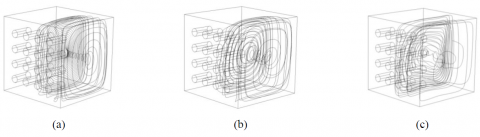Figure 2. Some particles trajectories for case 1 and b = 0.25 ; (a) Ra = 103; (b) Ra = 104; (c) Ra = 105

## 3.pngFigure 3. Iso-surfaces of temperature for case 1 and b = 0.25 ; (a) Ra = 103; (b) Ra = 104; (c) Ra = 105

Iso-contours of Nuloc on cold wall for case 1 and b = 0.25 are shown in Fig. 4, for different Rayleigh numbers. Due to domination of conductive heat transfer mode, Nuloc is presented almost horizontal at the central region of the wall. U-shaped distribution is observed at the top of the wall due to the existence of an intensive vertical air movement. Values of Nuloc decrease from top to bottom of the cold wall as an expected result.

## 4.png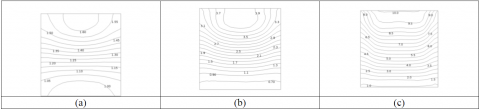Figure 4. Local Nusselt number at cold wall, for case 1 and b = 0.25; (a) Ra = 103; (b) Ra = 104; (c) Ra=105

Fig. 5 presents the variation of Nuav with Ra for case 1. As seen from the figure, Nuav is increased almost linearly with increasing of Ra. But values of Nuav are decreased with decreasing of b due to decreasing of incoming energy into system.

Total, friction and thermal local entropy generation are presented in Fig. 6 at z = 0.2 plan. Produced entropy due to heat transfer becomes higher near the edges of fins but the contours are denser near the bottom of the cavity due to rising of the air flow from bottom to top. This effect is clear for higher Rayleigh numbers as seen from the figures. Also, entropy is generated near the top right wall due to the clustering of temperature near that part as noticed from iso-surfaces of temperature. Entropy generation due to friction is presented in the second row of the Fig. 6 and it is noticed that it is concentrated near of the walls due to the manifestation of the viscous effect.

## 5.png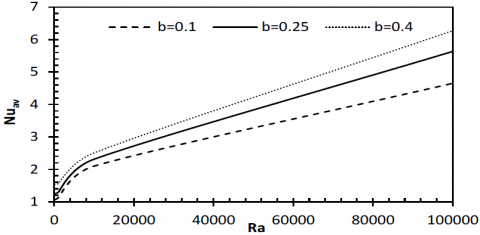Figure 5. Mean Nusselt number for case 1

## 6.png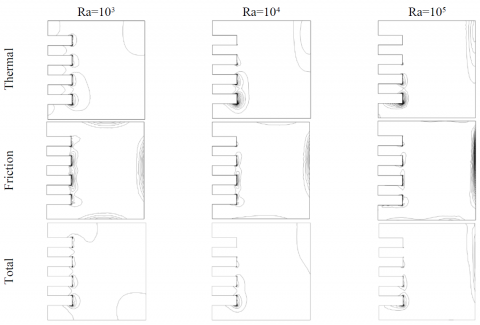Figure 6. Local entropy generation in z = 0.2 plan

## 7.png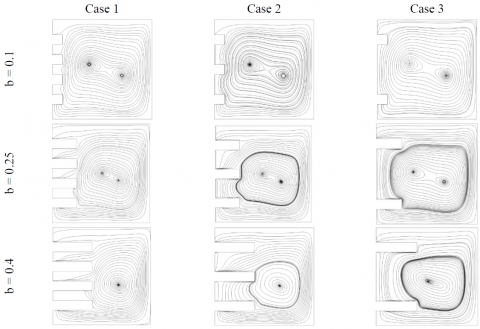Figure 7. Projection of the velocity vector in z = 0.2 plan for Ra = 105.

Entropy generation contours on walls becomes thinner with the increasing of Ra due to decreasing of boundary layer. Also, total entropy generation is presented on the bottom row and it presents similar distribution with other figures. As seen from the figures, edges of the pins are very effective on entropy generation.

Variations of thermal, viscous and total entropy generations are presented in Fig. 7 at different Rayleigh number for case 1. Entropy generation increases almost linearly with Ra due to incoming energy into the system.

For a best understanding of the flow structures vector velocity projections in z = 0.2 plan for Ra = 105 are presented in Fig. 7 for different lengths and numbers of fins. It is noticed that numbers and location of the vortex becomes same fit the same values of fin length. However, flow strength is a function of fin length.

Variations of Nuav as a function of length of fin for different cases are given in Fig. 8. As an expected result, heat transfer is decreased with decreasing of fin number, thus, heat transfer becomes lowest for case 3 and results for case 1 and 2 are almost the same. As a similar manner, total entropy generation is decreased with number of fin and maximum total entropy generation is observed for case 1 as given in Fig. 9. Total entropy generation value is increased with fin length due to increasing of heat transfer surface.

## 8.pngFigure 8. Average Nusselt number as function of b for Ra=105

## 9.pngFigure 9. Total entropy generation as a function of b for Ra=105

6. Conclusion

Effects of heated fin number, fin length and Rayleigh number on heat transfer, fluid flow and entropy generation are studied for three-dimensional domain by using a numerical technique. It is noticed that the main effective parameter on heat transfer, fluid flow and entropy generation are length and location of the fins. Edge of the fins plays the dominant role on entropy distribution due to flow friction at that part. Increasing of heat transfer and total entropy generation is almost linear with Rayleigh number. The minimum of produced entropy is observed for the highest number of fins, namely, case 1. Increasing of fin length enhances both heat transfer and entropy generation.

Nomenclature
 b Fin length g Acceleration due to gravity (m.s-2) k Thermal conductivity (W.m−1.K−1) l Enclosure Width lz Enclosure depth Nu Nusselt number Ns Local dimensionless entropy P Pressure (N.m-2) Pr Prandtl number $\overset{\scriptscriptstyle\rightharpoonup}{q}$ Heat flux vector Ra Rayleigh number S Generated entropy t Dimensionless time T Dimensionless Temperature V Dimensionless velocity vector Greek symbols α Thermal diffusivity (m2·s-1) β Thermal expansion coefficient (K-1) ΔT Temperature difference (K) μ Dynamic viscosity, (kgm-1 s-1) ν Kinematic viscosity (m2·s-1) ρ Density (kg·m-3) Φ’ Dissipation function φ Irreversibility coefficient $\psi$ vector potential $\omega$ vorticity Subscripts c cold f fluid fr friction gen generated h hot m average n normal th thermal tot total x, y, z Cartesian coordinates 0 Reference Superscript ' dimensionnal variable
References

 Incropera F.P. (1988). Convection heat transfer in electronic equipment cooling, J. Heat Transfer, Vol. 110, pp. 1097-1111. DOI: 10.1115/1.3250613

 Baskaya S., Erturhan U., Sivrioglu M. (2005). An experimental study on convection heat transfer from an array of discrete heat sources, Int. Comm. Heat Mass Transfer, Vol. 32, pp. 248-257. DOI: 10.1016/j.icheatmasstransfer.2004.03.018

 Huang G.J., Wong S.C., Lin C.P. (2014). Enhancement of natural convection heat transfer from horizontal rectangular fin arrays with perforations in fin base, Int. J. Thermal Sci., Vol. 84, pp. 164-174. DOI: 10.1016/j.ijthermalsci.2014.05.017

 Hassan A.R., Gbadeyan J.A. (2015). A reactive hydromagnetic internal heat generating fluid flow through a channel, International Journal of Heat and Technology, Vol. 33, No. 3, pp. 43-50. DOI: 10.18280/ijht.330306

 Bouchoucha A., Bessaïh R. (2015). Natural convection and entropy generation of nanofluids in a square cavity, International Journal of Heat and Technology, Vol. 33, No. 4, pp. 1-10. DOI: 10.18280/ijht.330401

 Bar-Cohen A., Iyengar M., Kraus A.D. (2003). Design of optimum plate-fin natural convective heat sinks, J. Heat Transfer, Vol. 125, pp. 208-216. DOI: 10.1115/1.1568361

 Kim S.J., Kim D.K., Oh H.H. (2008). Comparison of fluid flow and thermal characteristics of plate-fin and pin-fin heat sinks subject to a parallel flow, Heat Transfer Engineering, Vol. 29, pp. 169-177. DOI: 10.1080/01457630701686669

 Yalcin H.G., Başkaya S., Sivrioğlu M. (2008). Numerical analysis of natural convection heat transfer from rectangular shrouded fin arrays on a horizontal surface, International Communications in Heat and Mass Transfer, Vol. 35, pp. 299311. DOI: 10.1016/j.icheatmasstransfer.2007.07.009

 Varol Y., Oztop H.F., Yılmaz T. (2007). Natural convection in triangular enclosures with protruding isothermal heater, Int. J. Heat Mass Transfer, Vol. 50, pp. 2451-2462. DOI: 10.1016/j.ijheatmasstransfer.2006.12.027

 Appadurai M., Velmurugan V. (2015). Performance analysis of fin type solar still integrated with fin type mini solar pond, Sustainable Energy Tech. Assessments, Vol. 9, pp. 30-36. DOI: 10.1016/j.seta.2014.11.001

 Ryu K., Lee K.S. (2015). Generalized heat transfer and fluid flow correlations for corrugated louvered fins, Int. J. Heat Mass Transfer, Vol. 83, pp. 604-612. DOI: 10.1016/j.ijheatmasstransfer.2014.12.044

 Joo Y., Kim S.J. (2015). Comparison of thermal performance between plate-fin and pin-fin heat sinks in natural convection, Int. J. Heat Mass Transfer, Vol. 83 pp.345-356. DOI: 10.1016/j.ijheatmasstransfer.2014.12.023

 Baskaya S., Sivrioglu M., Ozek M. (2000). Parametric study of natural convection heat transfer from horizontal rectangular fin arrays, International Journal of Thermal Science, Vol. 39, pp. 797805. DOI:10.1016/S1290-0729(00)00271-4

 Da Silva A.K., Gosselin L. (2005). On the thermal performance of an internally finned three-dimensional cubic enclosure in natural convection, Int. J. Thermal Sci., Vol. 44, pp. 540-546. DOI: 10.1016/j.ijthermalsci.2004.11.011

 Bocu Z., Altac Z. (2011). Laminar natural convection heat transfer and air flow in three-dimensional rectangular enclosures with pin arrays attached to hot

wall, Appl. Thermal Eng, Vol. 31, pp. 3189-3195. DOI: 10.1016/j.applthermaleng.2011.05.045

 Kolsi L., Oztop H.F., Alghamdi A., Abu-Hamdeh N., Borjini M.N., Aissia H.B. (2016). A computational work on three-dimensional analysis of natural convection and entropy generation in nanofluid filled enclosures with triangular solid insert at the corners, Journal of molecular liquids, Vol. 218, pp. 260–274. DOI: 10.1016/j.molliq.2016.02.083

 Kolsi L., Mahian O., Oztop H.F., Aich W., Borjini M.N., Abu-Hamdeh N., Aissia B.H. (2016). 3D buoyancy induced flow and entropy generation of nanofluid filled open cavity having adiabatic diamond shaped obstacle, ENTROPY, Vol. 18, No. 6, pp. 232. DOI:10.3390/e18060232

 Kolsi L., Kalidasan K., Alghamdi A., Borjini M.N., Kanna P.R. (2016). Natural convection and entropy generation on a cubical cavity with twin adiabatic blocks and filled by aluminium oxide - water nanoﬂuid, Numerical Heat Transfer Part A, Vol. 70, No. 3, pp. 242–259. DOI: 10.1080/10407782.2016.1173478

 Kolsi L., Abu-Hamdeh N., Oztop H., Alghamdi A., Borjini M.N., Aissia H.B. (2016) Natural convection and entropy generation in a three dimensional volumetrically heated and partially divided cavity, International Journal of Numerical Methods for Heat & Fluid Flow, Vol. 26, No. 8, pp. 2492-2508. DOI: 10.1108/HFF-09-2015-0358

 Kolsi L. (2016). Numerical study of natural convection and entropy generation of Al2O3-water nanofluid within a cavity equipped with a conductive baffle, Journal of Applied Fluid Mechanics, Vol. 9, No. 5, pp. 2177-2186.

 Kolsi L. (2016). Numerical analysis of periodic 3D convective heat transfer in fenestration with between-the-glass louvered blinds, Case Studies in Thermal Engineering, Vol. 8, pp. 71-83, DOI: 10.1016/j.csite.2016.05.002

 Kolsi L., Oztop H.F., Abu-Hamdeh N., Alghamdi A., Borjini M.N. (2016). Three-dimensional analysis of natural convection and entropy generation in a sharp edged finned cavity, Alexandria Engineering Journal, Vol. 55, pp. 991–1004. DOI: 10.1016/j.aej.2016.02.030

 Bejan A. (1996). Entropy Generation Minimization, CRC Press, Boca Raton, FL.

 Wakashima S., Saitoh T.S. (2004). Benchmark solutions for natural convection in a cubic cavity using the high-order time–space method, Int. J. Heat Mass Transfer, Vol. 47, pp. 853–864. DOI: 10.1016/j.ijheatmasstransfer.2003.08.008

 Fusegi T., Hyun J.M., Kuwahara K., Farouk B. (1991). A numerical study of three-dimensional natural convection in a differentially heated cubical enclosure, Int. J. Heat Mass Transfer, Vol. 34, No. 6, pp. 1543–1557. DOI: 10.1016/0017-9310(91)90295-P